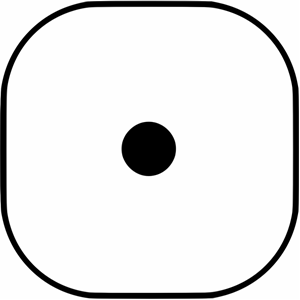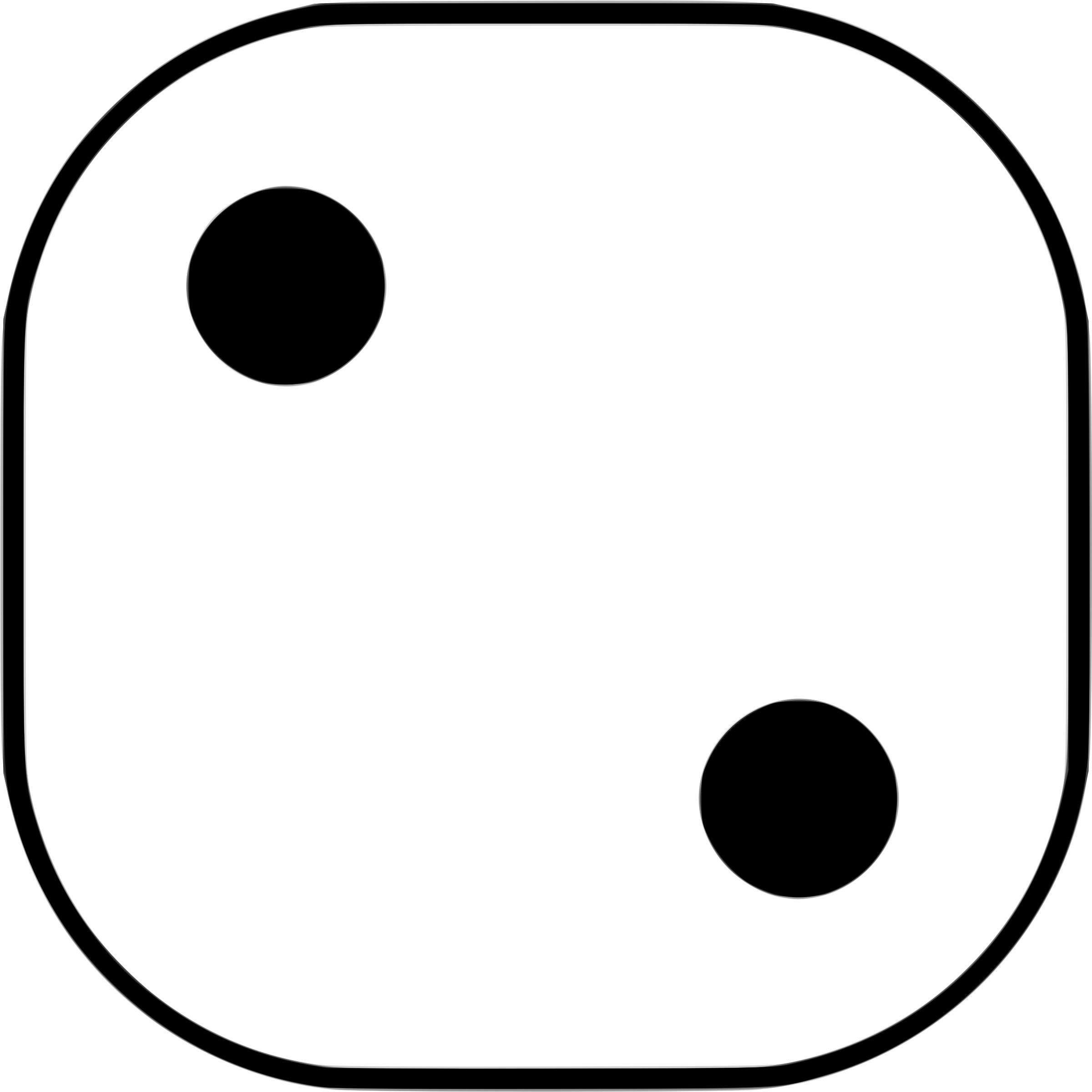## 16.7 Quick review questions

• Suppose Event A is defined as ‘I will roll aor aon a fair die.’
1. What is the best way to compute the probability of Event A occurring?
2. What is the probability of Event A occurring?
3. Suppose Event B is defined as ‘A randomly-chosen university student will like pizza.’ What is the best way to compute the probability of Event B occurring?
4. True or false: Events A and Event B are independent.
• Consider these three events, then answer the questions that follow:
• Event 1 is ‘The first card I pick from a standard 52-card pack will be an Ace’;
• Event 2 is ‘The second card I pick from a standard 52-card pack will be an Ace, if I do not return the first card’; and
• Event 3 is ‘The second card I pick from a standard 52-card pack is an Ace, if I do return the first card to a random location.’
1. True or false: Event 1 and Event 2 are independent.

2. True or false: Event 1 and Event 3 are independent.

3. The probability of Event 2 is: